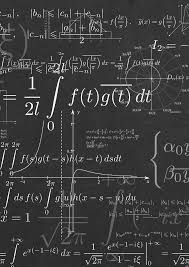Journal of Differential

Equations and Related

Fields

ISSN: Under Process

Editor

Prof.
Hugo Leiva (Ph.d)

Departamento de Matemáticas, Venezuela

E-mail:
hleivatoka@gmail.com

Subject Area: Mathematics (Differential Equations)

Published in UK
by Roman Science Publications Inc.ISSN: Under Process

Journal Metrics
Acceptance rate:  Not available
Submission to final decision:  Not available
Acceptance to publication:  Not available

Aim and Scope

Journal Differential Equations and Related Fields is an International peer reviewed journal. Journal Differential Equations and Related Fields, whose name is intended to cover the need of those researchers who work in the area of ​​differential equations and related fields. We know that when one wants to model a real-life problem, these problems are often modeled by differential equations, equations that involve an unknown function and its derivatives, but sometimes the differential equation is not enough to have a more accurate model. since the real problem is always subject to disturbances such as nonlocal conditions, impulses, delays, noises, etc. Therefore, we want a journal that includes among other topics:

1. Ordinary differential equations.

2. Partial differential equations.

3. Differential equations with impulses.

4. Differential equations with non-local conditions.

5. Differential equations with delays.

6. Fractional differential equations.

7. Differential equations on time scales.

8. Control systems governed by differential equations.

9. Optimal control problems.

8. Problems from mathematical biology represented by differential equations.

9. Dynamical systems with applications in differential equations.

10. Qualitative theory of dynamic systems and differential equations.

11. Numerical analysis for analyzing differential equations.

12. Evolution Equations.

13. Abstract differential equations in general.

14. Stochastic differential equations.

Submission: Authors  requested to submit their papers electronically to  hleivatoka@gmail.com

Article Processing Charges: No Charges for publication in the journal.